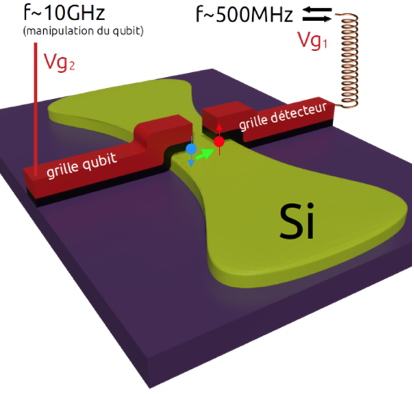highlight / actuality

# The spectrum of holes haunts qubitsUsually, quantum engineering designs quantum bits with semiconductor components, exploiting the properties of superconducting elements or electrons. In recent years, researchers at our laboratory have developed a very promising alternative by using holes instead of electrons.

Published on 20 December 2021
Today, most of the quantum bits (qubits) developed for quantum engineering are made with superconductors or with electrons in semiconductors. With the same electronic components in silicon, the usual material of classical transistors, but by miniaturizing them to the extreme (width and length of about 50 nm), researchers have designed excellent quantum dots that operate at very low temperatures (less than 100 mK). These quantum dots allow the isolation of a single charge (electron or hole). The spin carried by this charge constitutes the qubit.

Usually, the spin is manipulated with a radio frequency magnetic field (like in NMR for example). In recent years, researchers at our laboratory have developed a very promising alternative by using holes rather than electrons because, in the case of silicon, the spin of holes can be coupled with a radio frequency electric field. Thus, this coupling is simpler to realize experimentally since it is enough to apply a radio frequency voltage on the gate of the transistor.
To have a functional spin qubit it is not sufficient to manipulate the spin, it is still necessary to be able to read its state. To do this, researchers use two coupled quantum dots where the second dot serves as a spin detector, using the Pauli principle which requires that two spins on the same energy level must be opposite. In practice, the manipulation of the spin as well as its readout requires the application of precise voltages, but which are unknown a priori. It is therefore necessary to finely characterize the energy spectrum of the qubits in order to be able to apply the right voltages to them. For this, the researchers use a two-frequency spectroscopy technique.

These results make it possible to adjust the operating parameters of the "all-electric" spin qubit for the continuation of the projects in progress at IRIG, in particular for larger scale quantum processors.Schematic diagram of the silicon nanowire between two transistor gates. Under each gate, a hole is trapped. The spin of this hole is for example "up" (red arrow) or "down" (blue arrow). The spin can be transferred from one gate to the other (green arrow) by applying the right voltages. A 10 GHz signal is applied to rotate the spin in the "qubit" dot. In the other dot, the spin state is detected by a resonator at the resonance frequency of 500 MHz.

To go further:
To obtain the energy spectrum of qubits, the researchers apply a two-frequency spectroscopy technique.
A first signal at high frequency (ten gigahertz) corresponds to the transition between the two states 0 or 1 of the qubit. This gives access to the g factor, known as the "Lande factor", which translates the relationship between the applied static magnetic field (B) and the energy (E), according to the formula E=gµB (where µ is a constant, called Bohr’s magneton).
The second signal allows to read very quickly (about 10 µs) the spin state thanks to the second dot (detector) and according to the technique called radiofrequency reflectometry. This is made possible by another resonance, created by the detector, and an inductance of a few hundred nH placed in series. By working at the resonance frequency (about 500 MHz), we become extremely sensitive to the transitions between the two boxes, especially by reading out the phase of the reflected signal.

In addition to the Lande factor, it is possible to determine the tunnel coupling between the dots, as well as the strength of the spin-orbit coupling specific to our hole qubits. These parameters allow in return to apply the right signals on the qubit, to optimize for example the coherence time.

Top page